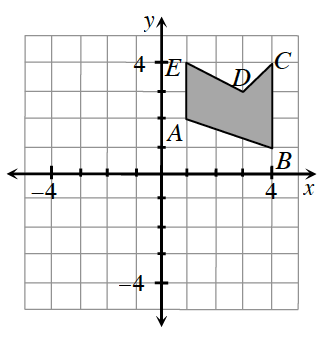### Home > GB8I > Chapter 11 Unit 12 > Lesson INT1: 11.2.3 > Problem11-88

11-88.Copy the graph below onto graph paper.

1. If the shape $ABCDE$ were rotated about the origin $180°$, where would point $A'$ be?

• $A'=(-1,-2)$

1. If the shape $ABCDE$ were reflected across the $x$-axis, where would point $C'$ be?

$C'=(4,-4)$

2. If the shape $ABCDE$ were translated so that each point $\left(x, y\right)$ corresponds to $\left(x-1,y+3\right)$, where would point $B'$ be?

Move left $1$ and up $3$.

$B'=(3,4)$